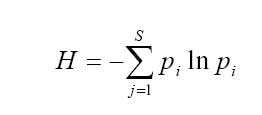CSIR NET June 2018
Online Exam
mc2exam.com
Quick Enquiry

# Calcualtion of Shannon Diversity indexOct 25,2015

From ecology section the questions you get in CSIR exam are of generally two types. First either in graphical representation or second based upon some formulae.Here we will be discussing one of the topic from ecology- Calculation of Shannon diversity index

A diversity index is a quantitative measure that reflects how many different types  there are in a dataset, and simultaneously takes into account how evenly the basic entities (such as individuals) are distributed among those types. The value of a diversity index increases both when the number of types increases and when evenness increases. For a given number of types, the value of a diversity index is maximized when all types are equally abundant.

Shannon Diversity Index

s

H = ∑ - (Pi * ln Pi)

i=1

where:

H = the Shannon diversity index

Pi = fraction of the entire population made up of species i

S = numbers of species encountered

Note:  The power to which the base e (e = 2.718281828.......) must be raised to obtain a number is called the natural logarithm (ln) of the number.

E,g.

The following table shows no of individuals of each species found in two communities

 Species B D C1 25 25 25 25 C2 80 05 05 10

(Hint: ln value for 0.05, 0.10, 0.25 and 0.80 are -3.0,-2.3,-1.4 and

-0.2respectively)

Calculated Shannon diversity index (H) value for C1 and C2 respectively are

1. 1.4 & 0.69
2. 1.2  & 0.34
3. 2.1  &0.43
4. 2.1 &0.3

Ans:

 Pi value for C1 ln of pi (Pi * ln Pi) 25/100=0.25 -1.4 0.25*-1.4=-0.35 25/1000=0.25 -1.4 0.25*-1.4=-0.35 25/1000=0.25 -1.4 0.25*-1.4=-0.35 25/1000=0.25 -1.4 0.25*-1.4=-0.35

s

H = ∑ - (Pi * ln Pi)

i=1

=∑-(-1.4)

=1.4

 Pi value for C2 ln of pi (Pi * ln Pi) 80/100=0.8 -0.2 0.80*-0.2=-0.16 0.5/100=0.05 -3.0 0.05*-3.0=-0.15 0.5/100=0.05 -3.0 0.05*-3.0=-0.15 10/100=.10 -2.3 0.10*-2.3=-0.23

s

H = ∑ - (Pi * ln Pi)

i=1

=∑-(-0.69)

=.69